Reach Us+44-1522-440391
Inference and Sample Size Calculations Based on Statistical Tests in a Negative Binomial Distribution for Differential Gene Expression in RNAseq Data
Journal of Biometrics & Biostatistics

Like us on:

All submissions of the EM system will be redirected to Online Manuscript Submission System. Authors are requested to submit articles directly to Online Manuscript Submission System of respective journal.

# Inference and Sample Size Calculations Based on Statistical Tests in a Negative Binomial Distribution for Differential Gene Expression in RNAseq Data

Xiaohong Li1,2, Nigel GF Cooper2, Yu Shyr3, Dongfeng Wu1, Eric C Rouchka4, Ryan S Gill5, Timothy E O’Toole6, Guy N Brock1,7 and Shesh N Rai1*

1Department of Bioinformatics and Biostatistics, University of Louisville, Louisville, KY, 40202, USA

2Department of Anatomical Sciences and Neurobiology, University of Louisville, Louisville, KY, 40202, USA

3Department of Biostatistics, Vanderbilt University, Nashville, TN, 37232, USA

4Department of Computer Engineering and Computer Science, University of Louisville, Louisville, KY, 40292, USA

5Department of Mathematics, University of Louisville, Louisville, KY, 40292, USA

6Department of Cardiology, University of Louisville, Louisville, KY, 40202, USA

7Department of Biomedical Informatics, Ohio State University, Columbus, OH, 43210, USA

*Corresponding Author:
Rai SN
Department of Bioinformatics and Biostatistics
University of Louisville Louisville
KY, 40202, USA
Tel: 1-502-852-4030
E-mail: [email protected]

Received Date: December 21, 2016; Accepted Date: January 25, 2017; Published Date: January 30, 2017

Citation: Li X, Cooper NGF, Shyr Y, Wu D, Rouchka EC, et al. (2017) Inference and Sample Size Calculations Based on Statistical Tests in a Negative Binomial Distribution for Differential Gene Expression in RNA-seq Data. J Biom Biostat 8: 332. doi:10.4172/2155-6180.1000332

Copyright: © 2017 Li X, et al. This is an open-access article distributed under the terms of the Creative Commons Attribution License, which permits unrestricted use, distribution, and reproduction in any medium, provided the original author and source are credited.

Visit for more related articles at Journal of Biometrics & Biostatistics

#### Abstract

The high throughput RNA sequencing (RNA-seq) technology has become the popular method of choice for transcriptomics and the detection of differentially expressed genes. Sample size calculations for RNA-seq experimental design are an important consideration in biological research and clinical trials. Currently, the sample size formulas derived from the Wald and the likelihood ratio statistical tests with a Poisson distribution to model RNA-seq data have been developed. However, since the mean read counts in the real RNA-seq data are not equal to the variance, an extended method to calculate sample sizes based on a negative binomial distribution using an exact test statistic was proposed by Li et al. in 2013. In this study, we alternatively derive five sample size calculation methods based on the negative binomial distribution using the Wald test, the log-transformed Wald test and the log-likelihood ratio test statistics. A comparison of our five methods and an existing method was performed by calculating the sample sizes and the simulated power in different scenarios. We first calculated the sample sizes for testing a single gene using the six methods given a nominal significance level α at 0.05 and 80% power. Then, we calculated the sample sizes for testing multiple genes given a false discovery rate (FDR) at 0.05 and 0.10. The empirical power and true prognostic genes for differential gene expression analysis corresponding to the estimated sample sizes from the six methods are also estimated via the simulation studies. Using the sample size formulas derived from log-transformed and Wald-based tests, we observed smaller sample properties while maintaining the nominal power close to or higher than 80% in all the settings compared to other methods. Moreover, the Wald test based sample size calculation method is easier to compute and faster in an RNA-seq experimental design.

#### Keywords

FDR; Sample size; Wald test; Exact test; Likelihood ratio test

#### Introduction

Sample size calculations are a prerequisite in an experimental design for biological research and clinical trials. Recently, highthroughput RNA sequencing (RNA-seq) technology has been widely used for gene expression studies in a variety of applications, such as expression profiling of mRNAs or non-coding RNA [1-3], de novo assembly and characterization of transcriptomes [4,5], and the identification of novel alternatively spliced transcript [6,7]. These novel transcripts or differentially expressed genes (DEGs) identified from RNA-seq data may serve as human disease biomarkers or gene signatures for the clinical diagnosis [8-10]. With the rapid growth of RNA-seq applications, sample size calculation methods derived from test statistics with an appropriate distribution are important issues to be explored and discussed.

Due to the initial high cost of RNA-seq, sample size, in terms of the number of biological replicates, was not seriously considered as part of the experimental design. As a case in point, one RNA-seq review article  documented several RNA-seq studies showing that many had only one or a few biological replicates. While thousands of DEGs were identified within these studies, the lack of biological replicates leads to an absence of knowledge concerning biological variations and may result in a high percentage of false positive genes. Therefore, ignorance of biological variation is the fundamental problem with the analyzed results collected from un-replicated data. A recent paper  was the first to point out that conclusions drawn from un-replicated samples can be misleading and unrealistic. Later, another study  further addressed design and validation issues due to the lack of biological replicates in RNA-seq data.

One of the key questions in an experimental design is to determine the number of biological replicates needed for differential expression analysis in order to achieve a desired statistical power given a significance level α and an underlying distribution. Since RNA-seq data are read counts, a Poisson distribution is commonly used as the model for identifying DEGs in RNA-seq data [14,15]. Fang and Cui were the first one to derive the sample size calculations based on a Wald statistical test with a Poisson distribution for single gene in RNA-seq. Later, several sample size calculation methods that were derived from the score statistic and the log-likelihood ratio test (LRT) statistic using the Poisson distribution were proposed . However, the assumption of a Poisson distribution that the expected mean and variance are equal usually does not hold for RNA-seq studies, where the variance is typically greater than the mean of the read counts . Therefore, a negative binomial distribution with a dispersion parameter is used to model RNA-seq data by the existing software packages such as DESeq  and edgeR , in which an exact test is used to test DEGs between conditions. Subsequently, a sample size calculation method based on an exact test statistic with the aid of the edgeR package  was proposed . However, sample size methods derived from other test statistics such as the Wald test, the LRT and an extension of Wald test via log-transformation using negative binomial distribution to model the RNA-seq data have not yet been explored.

Like microarray data, an RNA-seq dataset contains thousands of genes to be tested simultaneously and independently for differential expression analysis. A method for the adjustment or correction of p-values is required to control the type I error rate when multiple pairwise comparisons are performed. Instead of setting the critical value α at 0.05 or 0.01 for significance, a much lower critical value α* is required to correct for the inflation of α. The most common method to control the family-wise error rate (FWER) is the Bonferroni correction in which the adjusted p-value is computed via dividing the critical p-value by the total number of comparisons being made. The other widely used method for this multiple correction problem is an FDR correction . Since the Bonferroni correction with a large number of tests is more conservative than the FDR correction, using the Bonferroni correction results in a cost of increasing the probability of producing false negatives and consequently reducing the statistical power. For high dimensional microarray data analysis, an extension of the FDR correction was proposed  and is widely used by many researchers. To address similar issues for high-dimensional RNAseq data, a sample size determination based on the extended FDR correction from microarray data analysis was further proposed .

Our study is motivated by exploring sample size calculations using the well-known test statistics (the Wald test and LRT) and a negative binomial distribution to model RNA-seq data. In Section 2, we first define the Wald test, the log-transformed Wald test and the LRT statistics using the negative binomial distribution to model a single gene in RNA-seq data. Then, we derive sample size calculations based on these defined statistical tests. Lastly, we derived sample size calculation methods for testing multiple genes while controlling the FDR . In Section 3, we simulated power for testing single gene and multiple genes corresponding to the sample sizes estimated from our proposed methods and an existing method. The performance of these six methods is compared and evaluated via the required sample sizes and the estimated power. An application of real RNA-seq data to illustrate sample size calculations is presented in Section 4. Finally, we end with a discussion and conclusion in Section 5.

#### Methods

Derivation of test statistics

In an RNA-seq experiment, the data contains thousands of genes (g=1,…,G) with different number of reads for each sample mapped to the reference genome. Since the total number of reads among samples is different, the distribution of the gene in the sample with the same condition is not identical. A normalization factor called the size factor is used to model RNA-seq data with a negative binomial distribution For simplicity, the following statistical tests and consequent sample size calculation methods are based on a single gene tests for DEG analysis.

For a single gene in RNA-seq data, suppose that, for each condition i (I=0,1), the observations Xij (j=1,…,ni), are independent and identically follow a negative binomial distribution as Xij ~ NB (sij γi,Ï�) [17,22]. Under this setting, γi is the true gene expression level in condition i, sij is a size factor to normalize the raw read for the total number of reads mapped in the sample j, and Ï� is a dispersion parameter with the assumption Ñ�i=Ñ�. Thus, the summation of reads per gene per condition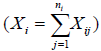also follows a negative binomial distribution with parameters nui=ti=si γi and Ñ� /n, where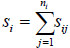is the summation of the size factor for mapping reads in condition i and n is the number of biological replicates with the assumption ni=n.

For detection of a differentially expressed gene from RNA-seq data, the ratio γ1/γ0 typically represents the fold change (ρ=γ1/γ0). If the fold change equals one, we can say that this gene is not differentially expressed. Therefore, we are interested in making an inference about the ratio using the Wald statistics and the likelihood ratio methods for sample size calculations.

For testing the hypothesis about the fold change in regards to the DEGs, it is equivalent to test the hypothesis,

H011 vs.H11 ≠ γ0 (1)

Since γi (i=0,1) and Ñ� are unknown parameters, we use the following two sample estimates for these parameters under the negative binomial distribution in RNA-seq data .

Unconstrained maximum likelihood estimate (MLE)

The likelihood and log-likelihood function of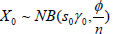and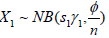are: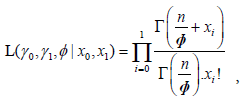(2)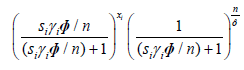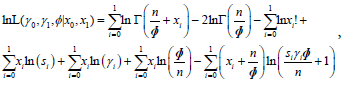(3)

Where t0=nu0=s0 γ0 and t1=nu1=s1 γ1.

Setting the first derivative to zero, we obtain the unrestricted MLEs with respect to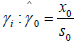and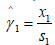. Subsequently, we can obtain the MLE of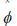by solving the following equation: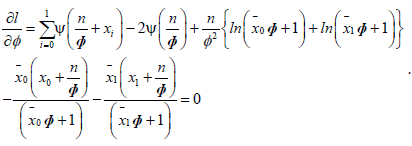(4)

Several mathematical optimization methods, such as the Newton- Raphson method, can be used to estimate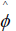for equation (4). Since there is no closing form to estimate the dispersion Ï�, we derived the sample size formula based on a constant value of Ï� estimated from the data.

Constrained maximum likelihood estimate (CMLE) for γi and Ñ�

The parameters are estimated under the null hypothesis H0: γ0=γ1.

Let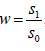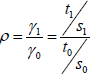and t1=ρwt0=ρwnu0. Then, the log likelihood function from equation (2) is re-parameterized as: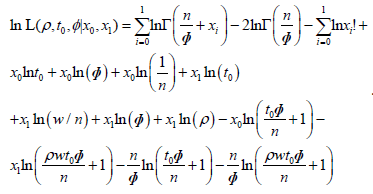(5)

Using partial derivatives of the equation (5) with respect to ρ and t0, the MLEs in the unrestricted parameter space are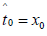and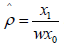. However, the CMLE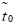in the constrained parameter space and under H0: ρ=1 is given by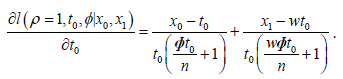(6)

Setting equation (6) to zero, we obtain: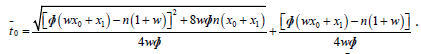(7)

Thus, setting the derivative of Ñ� to the zero and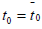and ρ=1, the CMLE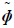for Ñ� is estimated by solving the following equation: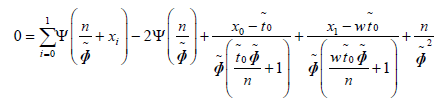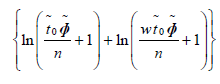We finally obtain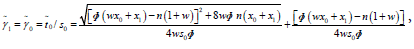(8)

and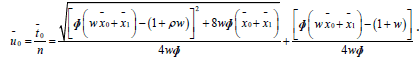(9)

Since there is no closing form to estimate the dispersion Ï� from MLE and CMLE, we derived the sample size formula based on a fixed and constant value. For simplicity, we set the dispersion in MLE and CMLE to be equal with a combination of fixed dispersion (0.1, 0.5 and 1).

Wald statistical test and log-transformed Wald statistical test

Wald statistical test: The Wald statistical test is an asymptotic test based on the normal approximation, which utilizes the large-sample properties of the MLE. Following procedures from the studies [24-26] for comparing two independent Poisson rates with unequal sample frames, we derived the Wald’s inference procedures using the properties of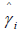and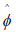estimated from MLE and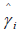and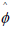from CMLE with a negative binomial distribution in two conditions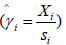, where X0 and X1 in two conditions are assumed to be independent. For simplicity, we set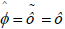with a constant for the sample size and power analysis.

The null hypothesis H0 in equation (1) is equivalent to H0: γ1 - γ0=0:, and consequently we make inferences based on the quantity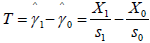. In this case, the variance of T is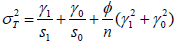and can be estimated by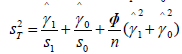, where the parameters are estimated form MLE. Thus, Wald statistical test from MLE can be obtained by the statistic T/ST: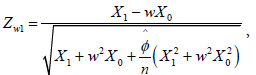(10)

Where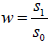is the ratio of total size factors between the two conditions.

Similarly, for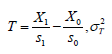can be estimated by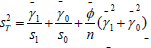using the parameters estimated from CMLE. By substituting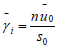from equation (8-9), the 2nd Wald statistical test can be obtained by the statistic T/ST: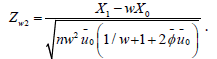(11)

Log-transformation of Wald’s statistical test: To test the null ypothesis, it is also equivalent to test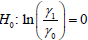. The logarithmic transformation is usually adopted for skewness correction and variance stabilization as suggested by these studies [16,24]. The statistical inference on the quantity is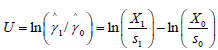. Since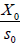and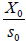have asymptotically normal distributions,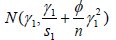and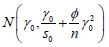, respectively,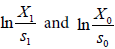correspondingly also have an asymptotically normal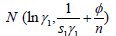and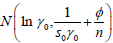by the Delta Method and Slutsky’s theorem. Thus, the variance of U is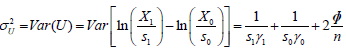U/SU can be used for testing H0, when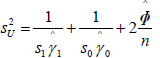using the parameters estimated from MLEs. Thus, a log-transformation of Zw1 is applied and the test statistic is defined as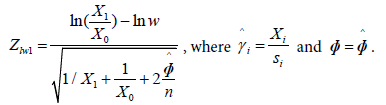(12)

Similarly, U/SU can be used for testing H0, when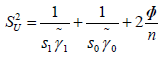using the parameters estimated from CMLE and then, we apply the log-transformation of Zw2 and use the test statistic: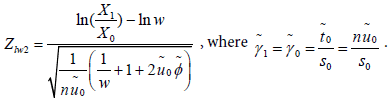(13)

We note that the equations defined in (12) and (13) do not exist when X0=0 or X1=0. In this case, X0 or X1 was adjusted to 0.5 [24,25].

Generalized likelihood ratio test (GLRT)

The GLRT statistic is defined as the ratio of the maximum value of the likelihood function under the restriction of the null hypothesis to the maximum likelihood function under the unrestricted parameter space. For a vector of parameters θ∈Θ, the GLRT for H0: θ∈Θ0 versus H1: θ∈Θ1 is expressed as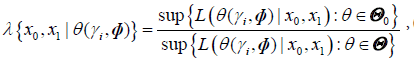(14)

Where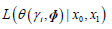is the likelihood function defined. The denominato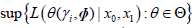in equation (14) is obtained using the MLE of θ, where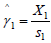and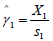. The numerator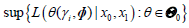is obtained using the CMLE of θ under H0, where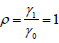. So the GLRT statistic is defined as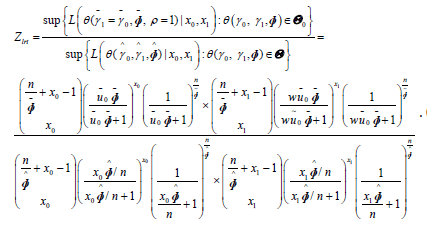(15)

Since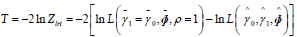approximately follows a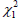distribution, the p-value is approximately: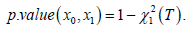(16)

The p-value in equation (16) is further adjusted by the FDR correction when multiple genes are used for the data analysis. Combining these together, the parameter estimates based on the MLEs from two assumptions and the following test statistics are summarized in Table 1.

Statistic tests Maximum Likelihood estimates (MLE) Statistical test Log Transformed test
Wald test MLE under unrestricted parameter space Zw1 Zlw1
Conditional MLE (CMLE) under H0: γ01 Zw2 Zlw2
Generalized Likelihood ratio test CMLE/MLE Zlrt

Table 1: Statistical tests are used for deriving sample size calculations. The parameters are estimated using MLEs and CMLE methods.

Sample size calculation for a single gene

In order to calculate the sample size, a power function needs to be constructed. The power of a test is the probability that the null hypothesis is rejected when the alternative hypothesis is true. We derive the sample size under the specified power 1-β and the significance level α with an assumption of a balanced design experiment between conditions (i.e., n0=n1=n) and one-sided statistical test under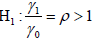Derivation of sample size based on the Wald test statistics: The details of derivation for each formula per test statistic are described in the Appendix: Derivation of sample size calculation in Supplementary Information. Briefly, given the parameters (u0 and Ï�), the sample size formula based on the Wald test statistics (Zw1,Zlw1,Zw2 and Zlw2) are defined as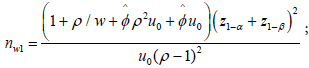(17)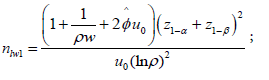(18)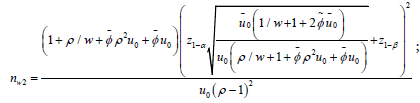(19)

And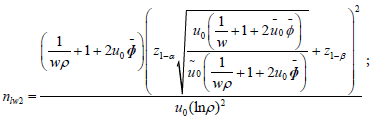(20)

Where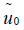is estimated from CMLE under H0 in equation (9) and u0 is the mean read counts under H1 or the assumed true mean read counts in the control condition. Under the alternative hypothesis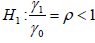Derivation of sample size based on the likelihood ratio test (LRT) statistic: For the LRT with a negative binomial distribution, it is difficult to derive a closed-form expression of the power function. We used the method  to calculate the power of the LRT given a p-value from the equation (16) under LRT. This method originally borrowed a concept from this study  to calculate the power. Given a p-value based on the observed joint probability P (X0=x0, X1=x1), the power under the assumption can be expressed as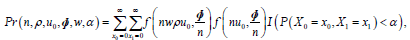(21)

where X0 and X1 are independent, f(u,Ñ�) is the probability mass function of the negative binomial distribution with mean u and dispersion Ñ�, α is the level of significance, and I(·) is the indicator function of p-value. Thus, given a nominal power 1-β, the power of the test can be represented as the function of the sample size n in the form of: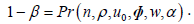(22)

Therefore, the required sample size n to attain the nominal power 1-β at a significance level α can then be computed by solving equation (22) through a numerical approach with respect to n.

Sample size calculation with controlling FDR for testing multiple genes

In an RNA-seq experiment, thousands of genes need to be tested simultaneously for DEGs between conditions. In this case, the sample size calculation for a single gene derived above needs to be further adjusted due to the multiple testing problems. In this section we derive sample size calculations by incorporating FDR controlling based on the statistical tests described in the previous sections. The details of controlling FDR have been given in the study . Briefly, FDR (f) is defined as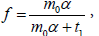(23)

Where m0 is the number of true null hypotheses, t1=E(M1) is the expected number of true rejections, M0 is the number of false discoveries, M is the total number of genes declared significant, M1=M-M0 and f is the control FDR at a specified level.

By solving equation (23) with respect to α, the marginal type I error level α* for the expected number of true rejections t1 at a given FDR (f) is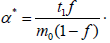(24)

Replacing α with α* in equation (24) in equations (17-20), the corresponding sample size calculation formulas corrected by FDR at level f, respectively,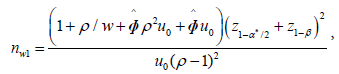(25)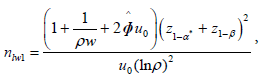(26)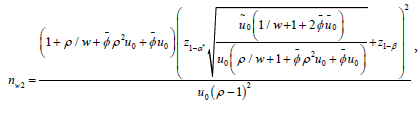(27)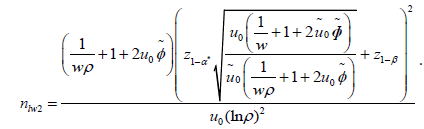(28)

Similarly, replacing α with α* for the LRT statistic in equation (22), we obtain the function with respect to n as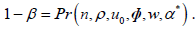(29)

Thus, by solving (29) via a numerical approach, the sample size for controlling FDR at level f can be obtained.

#### Simulation Studies and Comparison of Results

The proposed sample size formulas are derived from the likelihood function based on large sample theory with an approximate normal distribution. The simulation studies include two parts. In the first part, we calculated sample size based on testing single gene from the different formulas. In the second part, we calculated sample size based on testing the multiple genes using FDR adjusted significance α* level. The parameter settings in our simulation studies are based on empirical data sets. A comparison of the simulated power for the sample sizes using different methods was performed.

Sample size calculations and power estimation based on testing a single gene

The purpose of this study is to compare the performance of sample size calculations with the estimated power from our formula with the method based on the exact test in the public study . We set the following inputs based on a single gene. Let the type I error rate α=0.05, the power1-β=0.8, the ratio of total size factors between two condition w=1 and 1.2, the mean counts of gene g in control condition u0=1, 5 or 10, the dispersion Ñ�=0.1, 0.5 or 1, and the fold changes ρ=1.5, 2, 3 or 4. Since the read depth across samples in RNA-seq data is usually close to each other, we choose w=1.2 instead of w=2 . For each combination of these designed settings, at first, we used our derived formulas in equations (17-20 and 22) to calculate the required sample size, respectively. Then, we calculated the sample size based on the exact test computed using the R codes with the same input settings. Moreover, for each designed setting, we generated 5000 simulations from independent negative binomial distributions based on the calculated sample size n given the dispersion Ñ� with different mean counts. For the control condition (i=0), we used R to generate random samples given the mean u0 and Ñ�. For the treatment condition (i=1), we generated random samples given mean u1=wρu0 and Ñ�. The test statistics in equations (10-13 and 14-16) were applied to each simulation sample and the empirical power was obtained as the proportion of simulation samples for which H0 is rejected with the nominal type I error α=0.05. The results are shown in which reports the estimated sample size with associated empirical power given in parentheses under the case w=1 and 1.2.

Sample size calculation based on testing multiple genes via FDR-controlling method

In this study, we evaluated the performance of the sample size methods based on testing the multiple genes via FDR-controlling method rather than the type I error α, which is widely used in the RNA-seq analysis. We set m=10,000, m1=100, m0=m - m1 and want to detect the expected number of true DEG t1=80 and the actual power corresponding to the nominal power of 1-β=80%. We also set u0g=1,5 or 10, ρg=1.5,2,3 or 4 and Ñ�g=0.1,0.5 or 1.0. With these settings, the new α*=4.25 × 10-4 was obtained from the equation (24) at a desired FDR (f=0.05). Then, we calculated the sample size by substituting α*and power into the equations (25-29) and the published method using the exact test. For each designed setting, we also conducted 5000 simulations from an independent negative binomial distribution. The number of true DEGs was counted using p-values ≤ α* which is much smaller than the nominal type I error rate 0.05. The empirical power was obtained as the proportion of simulation samples for which H0 is rejected with the nominal type I error α*=4.25 × 10-4. The expected number of true DEGs under α* can be estimated via the multiplication of the estimated power with the total number of true DEGs. The results in Table 2 report the estimated sample size with the empirical power given in parentheses under the cases w=1 and 1.2.

w ρ Ñ� u0 nw1 nw2 nlw1 nlw2 nlrt nexact
1 1.5 .1 1 197 (0.80) 196 (0.81) 198 (0.81) 192 (0.80) 221 (0.82) 218 (0.80)
5 58 (0.81) 57 (0.81) 57 (0.81) 55 (0.79) 65 (0.84) 63 (0.81)
10 40 (0.81) 39 (0.80) 39 (0.80) 38 (0.79) 45 (0.84) 43 (0.80)
0.5 1 288 (0.81) 284 (0.82) 283 (0.81) 277 (0.80) 321 (0.83) 311 (0.80)
5 148 (0.82) 145 (0.81) 142 (0.80) 140 (0.80) 162 (0.83) 156 (0.81)
10 131 (0.82) 127 (0.80) 124 (0.81) 123 (0.80) 142 (0.83) 137 (0.81)
1 1 401 (0.81) 394 (0.81) 389 (0.81) 384 (0.80) 441 (0.82) 429 (0.80)
5 262 (0.82) 255 (0.81) 248 (0.80) 247 (0.80) 284 (0.83) 273 (0.80)
10 244 (0.83) 237 (0.82) 230 (0.81) 229 (0.81) 264 (0.84) 254 (0.81)
2 .1 1 61 (0.82) 60 (0.81) 62 (0.83) 57 (0.80) 67 (0.83) 67 (0.81)
5 19 (0.83) 18 (0.82) 18 (0.82) 17 (0.80) 21 (0.85) 20 (0.82)
10 14 (0.84) 13 (0.82) 13 (0.82) 12 (0.80) 15 (0.86) 14 (0.81)
0.5 1 96 (0.83) 92 (0.82) 91 (0.81) 86 (0.80) 101 (0.83) 99 (0.80)
5 54 (0.85) 51 (0.84) 47 (0.81) 46 (0.80) 54 (0.84) 53 (0.82)
10 49 (0.84) 45 (0.82) 42 (0.80) 41 (0.79) 48 (0.82) 47 (0.82)
1 1 140 (0.83) 133 (0.81) 127 (0.80) 122 (0.80) 143 (0.82) 140 (0.80)
5 98 (0.85) 91 (0.84) 84 (0.80) 83 (0.80) 96 (0.83) 93 (0.80)
10 92 (0.84) 85 (0.83) 78 (0.81) 78 (0.81) 90 (0.83) 87 (0.81)
3 0.1 1 22 (0.84) 21 (0.83) 22 (0.84) 18 (0.80) 23 (0.85) 23 (0.83)
5 8 (0.88) 7 (0.83) 7 (0.84) 6 (0.79) 8 (0.88) 8 (0.87)
10 6 (0.87) 5 (0.80) 5 (0.82) 4 (0.74) 6 (0.89) 6 (0.88)
0.5 1 39 (0.86) 36 (0.85) 34 (0.83) 30 (0.80) 36 (0.82) 37 (0.82)
5 25 (0.89) 22 (0.87) 18 (0.80) 18 (0.82) 21 (0.83) 21 (0.82)
10 24 (0.91) 20 (0.87) 16 (0.79) 16 (0.80) 19 (0.83) 19 (0.82)
1 1 61 (0.87) 54 (0.85) 48 (0.82) 44 (0.79) 54 (0.83) 53 (0.81)
5 47 (0.91) 40 (0.87) 33 (0.81) 32 (0.80) 38 (0.83) 37 (0.81)
10 45 (0.91) 38 (0.88) 31 (0.81) 30 (0.80) 36 (0.83) 35 (0.81)
4 0.1 1 13 (0.85) 12 (0.83) 13 (0.85) 10 (0.81) 13 (0.85) 13 (0.82)
5 5 (0.88) 5 (0.92) 4 (0.82) 3 (0.72) 5 (0.91) 5 (0.89)
10 4 (0.88) 4 (0.93) 3 (0.83) 3 (0.87) 4 (0.92) 4 (0.91)
0.5 1 26 (0.89) 23 (0.88) 20 (0.82) 17 (0.79) 22 (0.84) 22 (0.82)
5 18 (0.93) 15 (0.91) 11 (0.79) 11 (0.81) 13 (0.83) 13 (0.82)
10 17 (0.94) 14 (0.91) 10 (0.79) 10 (0.81) 12 (0.82) 12 (0.82)
1 1 43 (0.93) 36 (0.89) 30 (0.82) 26 (0.79) 33 (0.84) 33 (0.83)
5 35 (0.95) 28 (0.91) 20 (0.79) 20 (0.80) 24 (0.84) 24 (0.83)
10 34 (0.95) 27 (0.92) 19 (0.80) 19 (0.81) 23 (0.84) 23 (0.83)
1.2 1.5 0.1 1 180 (0.80) 184 (0.80) 186 (0.81) 177 (0.80) 205 (0.88) 202 (0.80)
5 54 (0.80) 55 (0.81) 54 (0.80) 52 (0.79) 62 (0.88) 60 (0.80)
10 38 (0.80) 38 (0.80) 38 (0.81) 37 (0.80) 43 (0.88) 42 (0.82)
0.5 1 270 (0.80) 273 (0.80) 271 (0.80) 262 (0.80) 306 (0.88) 296 (0.80)
5 145 (0.82) 143 (0.81) 139 (0.81) 138 (0.81) 159 (0.88) 153 (0.81)
10 129 (0.82) 126 (0.82) 123 (0.82) 122 (0.81) 140 (0.88) 135 (0.82)
1 1 384 (0.81) 383 (0.81) 377 (0.81) 369 (0.80) 427 (0.88) 413 (0.80)
5 258 (0.82) 253 (0.81) 245 (0.80) 244 (0.80) 279 (0.88) 269 (0.80)
10 243 (0.83) 236 (0.82) 229 (0.80) 228 (0.80) 260 (0.88) 251 (0.81)
2 0.1 1 55 (0.80) 57 (0.81) 59 (0.83) 52 (0.80) 62 (0.87) 62 (0.80)
5 18 (0.83) 18 (0.83) 18 (0.83) 16 (0.80) 20 (0.89) 19 (0.81)
10 13 (0.83) 13 (0.83) 12 (0.79) 12 (0.81) 14 (0.89) 14 (0.84)
0.5 1 90 (0.82) 90 (0.82) 88 (0.81) 82 (0.81) 97 (0.88) 95 (0.80)
5 53 (0.85) 50 (0.83) 47 (0.81) 45 (0.80) 53 (0.88) 52 (0.82)
10 48 (0.84) 45 (0.83) 41 (0.79) 41 (0.80) 48 (0.88) 46 (0.80)
1 1 134 (0.82) 130 (0.81) 124 (0.80) 118 (0.80) 139 (0.87) 136 (0.80)
5 97 (0.84) 90 (0.83) 83 (0.80) 82 (0.79) 95 (0.87) 92 (0.81)
10 92 (0.85) 85 (0.84) 78 (0.81) 77 (0.80) 89 (0.87) 86 (0.81)
3 0.1 1 20 (0.85) 20 (0.83) 21 (0.85) 17 (0.81) 21 (0.88) 22 (0.84)
5 7 (0.82) 7 (0.84) 7 (0.86) 6 (0.82) 7 (0.87) 7 (0.80)
10 6 (0.89) 5 (0.81) 5 (0.84) 4 (0.76) 6 (0.93) 6 (0.89)
0.5 1 37 (0.86) 35 (84) 33 (0.82) 29 (0.81) 35 (0.87) 35 (0.81)
5 25 (0.90) 22 (0.88) 18 (0.80) 17 (0.79) 21 (0.89) 21 (0.83)
10 23 (0.90) 20 (0.88) 16 (0.79) 16 (0.80) 19 (0.88) 19 (0.82)
1 1 59 (0.89) 53 (0.85) 47 (0.81) 43 (0.79) 53 (0.93) 52 (0.81)
5 47 (0.91) 40 (0.88) 33 (0.82) 32 (0.81) 38 (0.88) 37 (0.82)
10 45 (0.91) 38 (0.88) 31 (0.81) 30 (0.80) 36 (0.89) 35 (0.82)
4 0.1 1 12 (0.87) 12 (0.86) 13 (0.88) 9 (0.90) 12 (0.89) 12 (0.81)
5 5 (0.91) 4 (0.81) 4 (0.84) 3 (0.75) 5 (0.95) 5 (0.91)
10 4 (0.90) 4 (0.94) 3 (0.83) 3 (0.87) 4 (0.95) 4 (0.92)
0.5 1 25 (0.90) 22 (0.87) 20 (0.84) 16 (0.79) 21 (0.89) 21 (0.82)
5 18 (0.94) 15 (0.91) 11 (0.80) 11 (0.82) 13 (0.88) 13 (0.82)
10 17 (0.94) 14 (0.91) 10 (0.79) 10 (0.81) 12 (0.88) 12 (0.83)
1 1 41 (0.92) 35 (0.88) 29 (0.81) 26 (0.81) 32 (0.88) 32 (0.82)
5 35 (0.95) 28 (0.91) 20 (0.80) 20 (0.81) 24 (0.89) 24 (0.83)
10 34 (0.95) 27 (0.92) 19 (0.80) 19 (0.80) 23 (0.89) 22 (0.81)

Table 2: Simulated power for testing multiple genes with sample sizes. The sample sizes are calculated using six methods given a predefined FDR=0.05 and 80% power.

Given the sample size obtained from nlw2, an empirical power for other methods is also computed from 5,000 simulations (Table 3). In order to compare the performance of these methods the paired Wilcoxon signed-rank test is used to test the simulated power in Table 3 for the statistical significance. The results are in Table 4.

ρ Ñ� u0 nlw2 nlw1 nw1 nw2 nlrt nexact
w=1 1.5 0.1 1 192 (0.80) 0.79 0.79 0.79 0.74 0.72
5 55 (0.79) 0.78 0.78 0.78 0.73 0.72
10 38 (0.79) 0.78 0.77 0.78 0.73 0.72
0.5 1 277 (0.80) 0.79 0.79 0.78 0.73 0.72
5 140 (0.80) 0.79 0.78 0.78 0.74 0.73
10 123 (0.80) 0.80 0.78 0.80 0.75 0.74
1 1 384 (0.80) 0.80 0.78 0.78 0.74 0.74
5 247 (0.80) 0.80 0.78 0.79 0.74 0.73
10 229 (0.81) 0.81 0.79 0.80 0.75 0.74
2 0.1 1 57 (0.80) 0.77 0.77 0.78 0.73 0.70
5 17 (0.80) 0.78 0.76 0.78 0.73 0.71
10 12 (0.80) 0.78 0.74 0.77 0.72 0.71
0.5 1 86 (0.80) 0.78 0.76 0.77 0.73 0.71
5 46 (0.80) 0.79 0.74 0.77 0.73 0.72
10 41 (0.79) 0.78 0.72 0.76 0.72 0.72
1 1 122 (0.80) 0.78 0.74 0.77 0.73 0.71
5 83 (0.80) 0.79 0.73 0.77 0.73 0.72
10 78 (0.81) 0.81 0.74 0.78 0.75 0.74
3 0.1 1 18 (0.80) 0.70 0.71 0.71 0.70 0.64
5 6 (0.79) 0.74 0.66 0.73 0.69 0.66
10 4 (0.74) 0.70 0.53 0.65 0.63 0.61
0.5 1 30 (0.80) 0.74 0.66 0.73 0.70 0.66
5 18 (0.82) 0.80 0.63 0.75 0.74 0.72
10 16 (0.80) 0.79 0.59 0.73 0.72 0.71
1 1 44 (0.79) 0.76 0.62 0.72 0.70 0.67
5 32 (0.80) 0.79 0.57 0.73 0.72 0.71
10 30 (0.80) 0.79 0.55 0.72 0.72 0.71
4 0.1 1 10 (0.81) 0.65 0.62 0.71 0.67 0.62
5 3 (0.72) 0.62 0.39 0.58 0.58 0.53
10 3 (0.87) 0.81 0.62 0.78 0.78 0.76
0.5 1 17 (0.79) 0.71 0.52 0.67 0.67 0.63
5 11 (0.81) 0.79 0.41 0.69 0.71 0.70
10 10 (0.81) 0.79 0.33 0.68 0.71 0.70
1 1 26 (0.79) 0.74 0.44 0.66 0.67 0.64
5 20 (0.80) 0.79 0.34 0.68 0.71 0.69
10 19 (0.81) 0.80 0.30 0.68 0.72 0.71

Table 3: Simulated power for testing multiple genes given the sample size. The sample sizes are computed using nlw2 method at a predefined FDR=0.05 and 80% power.

nw1 nlw1 nw2 nexact nlrt nw1
nlw2 <0.0001* <0.0001* <0.0001* <0.0001* <0.0001*
nlw1 - <0.001* <0.0001* <0.0001* <0.0001*
nw2   - <0.001* <0.01* <0.05*
nexact     - <0.05* 0.8654
nlrt       - 0.5993

Table 4: P-values are calculated using the paired Wilcoxon signed-rank statistical test of the power values in Table 3.

Identify the pattern of the sample sizes changing with different values of u0, Ï� and ρ for different methods at α and α* levels

First, we identified patterns of sample size changes with different values of u0, Ï� and ρ for different methods. Although Table 2 show similar pattern of sample sizes obtained from the six methods, we found that the required sample sizes nlw1 and nlw2 derived from the log-transformed Wald tests are the smallest compared with the other methods.

Figure 1 illustrates nlw2 varying with the values of u0 when other parameters, such as ρ ∈ (1.5, 2, 3, 4), Ñ� ∈ (.1, .5, 1) and w ∈ (1, 1.2) are fixed. Given the nominal power (1-β=0.8) and α=0.05 or FDR=0.05, as expected, nlw2 decreases as u0 increases under a fixed ρ and Ñ�. This indicates that for a lowly expressed gene, a larger sample size is required to achieve a detection power of DEGs between two conditions. For a fixed u0 and ρ, nlw2 increases as Ï� increases (Figure 1). This is also expected because a larger Ï� indicates higher variation of the genes across conditions. Furthermore, for a fixed Ï� and u0, nlw2 decreases as ρ increases. This result indicates a smaller n is required for a larger difference of mean read counts between two conditions or vice versa. For the same setting of parameters, we found the nlw2 in Figure 1A and 1C with an equal size factor (w=1) across conditions is slightly larger than the unequal size factor in Figure 1B (w=1). Under the same settings, as expected, the sample sizes in Table 2 with FDR=0.05 are larger than those with α=0.05, indicating that a larger n is required for detecting DEGs while testing thousands of genes simultaneously (Figure 1C and 1D) compared with testing a single gene (Figure 1A and 1B).

Comparison of the sample calculations from different methods based on testing a single genes in multiple genes in Table 2

Figure 1: Sample size (n) for testing a single gene and multiple genes is computed using the nlw2 method. n varies with mean reads counts in control condition (u0)given the combination of fold change (ρ), dispersion (Ï�), and ratio of the size factors (w=1, 1.2) at 80% power and a nominal FDR (0.05) for testing a single gene (Figures 1A and 1B) or a nominal FDR (0.05) for testing multiple genes (Figures 1C and 1D). The fold changes (ρ=1.5, 2, 3 and 4) are in orange, green, blue and red, respectively.

Next we compared the sample sizes (n) estimated from our five derived methods (nw1, nw2, nlw1, nlw2, nlrt) and the public method (nexact) . Figure 2 illustrates that n decreases as u0 increases for all methods given w=1, power=0.8, FDR=0.05, Ñ� ∈ (0.1,0.5,1) and ρ ∈ (1.5,2). Given ρ=1.5, the sample sizes from all methods are getting close to each other when Ï� is 0.1 for small biological variation (Figure 2A- 2C). As Ï� increases, the difference between the sample sizes for all methods becomes much larger. Similar patterns were observed given ρ=2 (Figure 2D-2F). With regards to the n, we noticed that the sample sizes calculated from nlw1 and nlw2 methods (red in Figure 2) are the smallest while maintaining the nominal power close to or above 80%. Among the other four methods, no one performed better than others in all scenarios.

Figure 2: Sample size (n) for testing multiple genes is required using the six methods nw1, nw2, nlw1, nlw2, nlrt and nexact). n varies with mean counts in control condition (u0) given the combination of fold change (ρ) and dispersion (Ï�) at w=1, 80% power and 0.05 FDR. nlw1 and nlw2 methods in red and blue respectively require smaller n. Figures 2A and 2D illustrate samples sizes for all methods are close to each other when the dispersion Ï� is 0.1.

In addition, the empirical power in parentheses and Table 2 was calculated from simulations with the size of 5,000 corresponding to the estimated n for all methods. The results show almost all of the methods are close to or higher than the desired power. Although the sample sizes calculated using nlw1 and nlw2 are the smallest, we cannot arbitrarily conclude that these two methods are the best because the corresponding empirical powers are varied corresponding to the sample sizes for each method (Table 2).

For a better comparison with the same settings and a fixed and small n estimated from the log-transformed Wald method (nlw2), we observed that nlw1 and nlw2 consistently achieve a better power close to the nominal power 80% or higher in all scenarios compared to other methods (Table 3). We also observed that nlrt and nexact perform similarly and both of them achieve a higher power than nw1 when the fold change is great than 2. However, nw1 achieves a better power than nlrt and nexact when the fold change is at ρ ≤ 2 (Table 3). Table 4 from a paired Wilcoxon ranked test indicates that the empirical power from nlw2 is statistically significant from that achieved using other methods. Table 4 also shows that nlw1 performed significantly better than others four methods. Among these four methods, no one performed better than the others in all scenarios.

Application

Sample size calculation based on RNA-seq data in human breast cancer: To identify DEGs between two conditions, we explored a real human breast cancer dataset to calculate the sample size. Forty Estrogen receptor positive (ER+) and HER2 negative breast cancer primary tumors and 29 uninvolved breast tissue sample that were adjacent to ER+ primary tumors in .fastq format were downloaded from NCBI GEO (series ID GSE58135). The raw sequencing files were mapped to the human hg19 reference genome using tophat2 (v2.0.13) with bowtie version (2.2.3.0). The mapped counts for each gene per sample were then extracted using HTSeq-scripts-count (version 2.7). There are a total of 57,773 genes extracted. After filtering genes with the mean read counts less than one in two groups, 35,112 genes were left. These samples were loaded into edgeR to estimate common dispersion and size factors. With the aid of edgeR, the normalization factors called size factors are estimated using the “RLE” scaling factor method  and the estimated ratio of total size factors of the samples in each condition (w) is 1.1. The common dispersion Ï� is estimated as 0.48 using the CMLE method .

We assumed the top 400 of 35,122 genes (1.1%) are prognostic and have the largest fold changes. The minimum of average read counts among these genes in the control group served as pilot data was estimated as u0=1 . In addition, the sample sizes were estimated using u0=5,10 and 20. Suppose we want to set the nominal power to 80%, which indicates we want to identify 320 or more of the prognostic genes. Under the control FDR at f=0.10 and 80% power, we can set m=35,112, m1=400, m0=m-m1 and t1=320. The parameters ρg (g=1,…, 35, 112) were assumed to be unknown. Let the mean counts in the control condition u0g=1, 5, 10, or 20 and fold change ρg=1.5 or 2, 3 and 4 with common dispersion Ñ�g=0.48. With these settings, the new α*=9.992 × 10-4 was obtained from equation (24) at a desired FDR(f=0.10). Then, we calculated the sample size by substituting α*and the power into equations (25-29) for each method (Table 5).

ρ u0 nw1 nw2 nlw1 nlw2 nlrt nexact
w=1.1
Ñ�=.48
1.5 1 242 241 240 234 275 267
5 125 123 120 119 139 134
10 111 108 105 104 122 117
20 103 101 97 97 114 109
2 1 80 79 77 73 87 86
5 46 43 40 39 47 45
10 41 39 35 35 42 40
20 39 36 33 33 39 38
3 1 33 31 29 25 32 32
5 21 19 16 15 18 18
10 20 17 14 14 17 16
20 19 16 13 13 16 15
4 1 22 19 18 15 19 19
5 16 13 10 9 12 11
10 15 12 9 8 11 10
20 14 11 8 8 10 10

Table 5: The sample sizes per group required for a balanced design in human breast cancer RNA-seq data. The sample sizes are calculated given the nominal FDR=0.10 and 80% power.

Table 5 reports the samples sizes under different scenarios including various minimum mean read counts in control condition (1, 5, 10 and 20) and desired fold changes (1.5, 2, 3 and 4) while controlling the FDR at 0.10. We found that the original RNA-seq experiment  with a minimum sample size 31 in each condition can detect more than 80% of the prognostic genes at the FDR (f=0.10) and u0=1 if the desired fold change is 3 or more. Moreover, with a minimum sample size 42 in each condition, we found that it can detect more than 80% of the prognostic genes at the FDR (f=0.10) and u0=5 if the desired fold change is 2 or more.

#### Discussion and Conclusion

In this study, five methods (nw1, nw2, nlw1, nlw2, nlrt) were derived to calculate sample sizes using the Wald test and LRT statistics based on a negative binomial distribution for modeling an RNA-seq experiment. The parameters are estimated using the MLE and CMLE methods. Since the dispersion estimated from MLE has no closing form, it is difficult to derive the sample size formula. Therefore, all of the methods are based on a fixed and constant dispersion. A log-transformed approach corresponding to the modified Zw1 and Zw2 was used to derive two other test statistics (Zlw1 and Zlw2). For all these statistical tests as well as the exact test  that are used to derive the sample size calculation formulas, gene expression levels are assumed to be independent in each sample. Although this assumption might not hold in reality, it is widely used in RNA-seq as well as in microRNA data analysis. In this study, we assume equal sample size in the two conditions to derive the sample size formula. The derived formula for sample size calculations can be easily extended to the unequal sample sizes by setting n1=kn0. In our simulation study, we set the ratio of total size factors in two conditions as 1 and 1.2 instead of w=2 in the study . In reality, the read depths of RNA-seq samples generated from the same run are very close to each other across conditions. Therefore, we think w=1.2 or close to 1 is more common than w=2. Furthermore, in our simulation and application studies, the minimum sample sizes required to achieve a nominal power of 80% with a predefined FDR (f=0.05 or 0.10) are usually larger than those in an RNA-seq experiment due to the real costs. In such a situation, we can increase the read depth per sample to indirectly increase the mean of read counts u0 in the control condition. Thus, the required sample sizes can be decreased correspondingly.

Among the methods we evaluated, the simulation results show that nlw2 from the log transformed Wald test with the parameters estimated from CMLE is the best method because a smaller sample size is required for designing an RNA-seq experiment while achieving a power close to or higher than 80% at a pre-defined FDR=0.05. The second best method is nlw1 with the parameters estimated from unrestricted MLEs. We also found that nlrt and nexact methods perform better than nw1 method based on the estimated power given the genes with a fold change >2. However, nw1 achieve a better power than nlrt and nexact given a fold change ≤2. In summary, nw1 , nw2, nlrt and nexact methods varied with different scenarios. Finally, since the log-transformed sample size calculation methods are more robust, simpler and require less time, we hope our tables can help and benefit for researchers and scientists in the design of RNA-seq experiments.

#### Acknowledgments

We thank Dr. Shyr and his coauthors for sharing their R codes with us that were used for comparison of our methods with theirs. This work was supported by P20GM103436 (N.G. Cooper, PI). Dr. Rai was supported by Wendell Cherry Chair in Clinical Trial Research in James Graham Brown Cancer Center (Dr. DM Miller, Director).

#### References

Select your language of interest to view the total content in your interested language

### Article Usage

• Total views: 1780
• [From(publication date):
February-2017 - Jul 21, 2019]
• Breakdown by view type
• HTML page views : 1631Can't read the image? click here to refresh